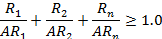TITLE 32: ENERGY
CHAPTER II: ILLINOIS EMERGENCY MANAGEMENT AGENCY
PART 337 PHYSICAL PROTECTION OF CATEGORY 1 AND CATEGORY 2 QUANTITIES OF RADIOACTIVE MATERIAL
SECTION 337.APPENDIX A CATEGORY 1 AND CATEGORY 2 RADIOACTIVE MATERIAL

Section 337.APPENDIX A   Category 1 and Category 2 Radioactive Material

Table 1 − Category 1 and Category 2 Threshold

The terabecquerel (TBq) values are the regulatory standard.  The curie (Ci) values specified are obtained by converting from the TBq value.  The curie values are provided for practical usefulness only.

 Category 1 Category 1 Category 2 Category 2 Radioactive material (TBq) (Ci) (TBq) (Ci) Americium-241 60 1,620 0.6 16.2 Americium-241/Be 60 1,620 0.6 16.2 Californium-252 20 540 0.2 5.40 Cobalt-60 30 810 0.3 8.10 Curium-244 50 1,350 0.5 13.5 Cesium-137 100 2,700 1 27.0 Gadolinium-153 1,000 27,000 10 270 Iridium-192 80 2,160 0.8 21.6 Plutonium-238 60 1,620 0.6 16.2 Plutonium-239/Be 60 1,620 0.6 16.2 Promethium-147 40,000 1,080,000 400 10,800 Radium-226 40 1,080 0.4 10.8 Selenium-75 200 5,400 2 54.0 Strontium-90 1,000 27,000 10 270 Thulium-170 20,000 540,000 200 5,400 Ytterbium-169 300 8,100 3 81.0

Note:   Calculations Concerning Multiple Sources or Multiple Radionuclides

The "sum of fractions" methodology for evaluating combinations of multiple sources or multiple radionuclides is to be used in determining whether a location meets or exceeds the threshold and is thus subject to the requirements.

I.          If multiple sources of the same radionuclide and/or multiple radionuclides are aggregated at a location, the sum of the ratios of the total activity of each of the radionuclides shall be determined to verify whether the activity at the location is less than the category 1 or category 2 thresholds of Table 1, as appropriate. If the calculated sum of the ratios, using the equation below, is greater than or equal to 1.0, then the applicable requirements apply.

II.        First determine the total activity for each radionuclide from Table 1. This is done by adding the activity of each individual source, material in any device, and any loose or bulk material that contains the radionuclide. Then use the equation below to calculate the sum of the ratios by inserting the total activity of the applicable radionuclides from Table 1 in the numerator of the equation and the corresponding threshold activity from Table 1 in the denominator of the equation. Calculations shall be performed in metric values (i.e., TBq) and the numerator and denominator values shall be in the same units.where:

 R1 = total activity for radionuclide 1 R2 = total activity for radionuclide 2 RN = total activity for radionuclide n AR1 = activity threshold for radionuclide 1 AR2 = activity threshold for radionuclide 2 ARN = activity threshold for radionuclide n

(Source:  Amended at 42 Ill. Reg. 42 Ill. Reg. 7485, effective April 4, 2018)